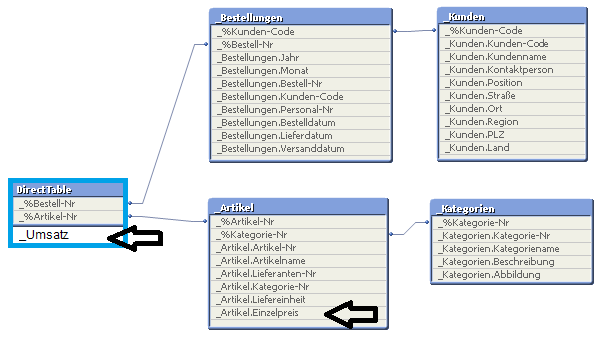# QlikView App Development

Discussion Board for collaboration related to QlikView App Development.

Announcements

#### Breathe easy -- you now have more time to plan your next steps with Qlik! QlikView 11.2 Extended Support is now valid through December 31, 2020. Click here for more information.Employee

## Use expressions with fields from both, Direct Table and In-Mem table

As you know, Direct Discovery operates with an SQL-based table. The "Direct Table" logic, that QlikView has since QV11.2 SR5, is aware of so-called DIMENSION fields, MEASURE fields and DETAIL fields (to be defined as such in the Load Script).

If QlikView finds inside of an expression a Measure field, it will look for the surrounding aggregation formula (SUM, AVG, MIN, MAX) and query that string (including the aggregation formula) to the SQL connection.

Assume that we have this structure. The DirectTable has one Measure Field (_Umsatz), which is not shown in the Table-Model Viewer, so I painted it there manually.If your expression is like:   SUM(_Umsatz)*0.8816  then SUM(_Umsatz) will be queried from SQL and the result will be multiplied with 0.8816 in QlikView.

In more detail: SUM(_Umsatz) will be in the middle of a SELECT statement, which also has a GROUP BY according to the current dimensionality context of the chart. There is likely also a WHERE clause if "_%Bestell-Nr" and "_%Artikel-NR" have any associated selection from any of the linked tables.

Now, assume you want to sum a datarow-wise multiplication of _Umsatz with another field from a linked in-memory table. If there was no Direct Table but a In-Memory-only data model, the formula would look like

SUM(_Umsatz * _Artikel.Einzelpreis)  ... this won't work in Direct Discovery. Why?

Because QlikView slices the expression into as many queries as it finds aggregation formulas and "roots" each part either to SQL or to the In-Memory Engine. The rule is, if one Direct Table Measure Field is involved in one aggregation formula, that entire aggregation formula is going to be sent to SQL. And it will fail here, since "_Artikel.Einzelpreis" is not a field in the DirectTable and unknown to SQL. QlikView can not mix two types of fields, In-Memory and SQL fields inside one Aggregation formula!

There is a way to solve this using Aggr() to create the dimensionality between the two linked tables (Direct Table and In-Memory) and then get the results:

Sum(Aggr(Sum(_Umsatz) * _Artikel.Einzelpreis, [_%Artikel-Nr]))

// don't judge this formula whether it makes business sense or not, it's just for illustration!

In the above example

• Aggr forms a temporary "hyper-cube" over the dimensionalty of "_%Artikel_Nr" which is the granularity of the linked table where my In-Memory field sits ("_%Artikel_Nr" is the primary key of the "Artikel" table).
• The Aggregation formula "Sum(_Umsatz)" is sent to, and successfully replied from, the SQL connection, grouped by "_%Artikel-Nr"
• Then the result-matrix, which has a compatible dimensionality now,  is multiplied with the *_Artikel.Einzelpreis".
• The outer Sum() is required if you use this formula in any other dimensionality than "Arikel" (example if this is in a chart where the dimensions is "_Kategorie.Kategoriename")

Don't use this too extensively, as the Aggr() enforces the Direct Discovery to make an addtional Query to SQL before it can continue with the calculation. This will have an effect on the performance of the chart generation.

>> Look into the options of "flattening" (joining) that desired field from the In-Memory table into the SQL table if possible

Enjoy Direct Discovery

Community Browser